Author: Oscar Cronquist Article last updated on May 23, 2022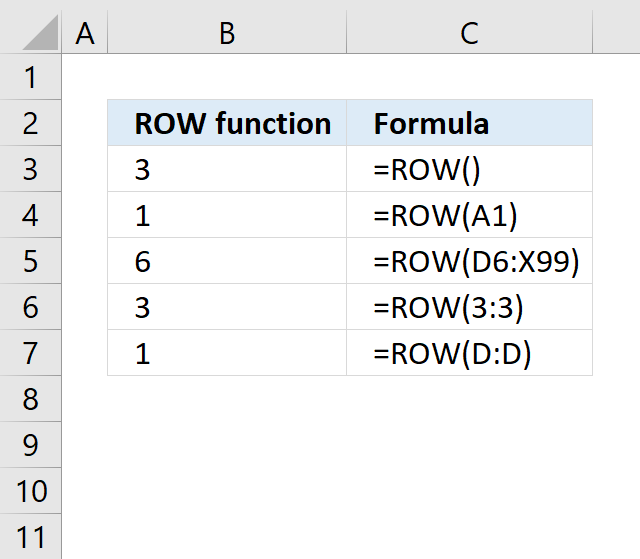The ROW function calculates the row number of a cell reference.

ROW(reference)

## 2. ROW Function Arguments

 reference Optional. A reference to a cell you want to know the row number of.

The ROW function is one of my most used Excel functions, that you probably already know if you have been browsing around my web site.

The first example demonstrates basic usage. Example 2 and 3 are more advanced and requires some knowledge about MATCH and INDEX function.

## 3. Example 1 - Cell references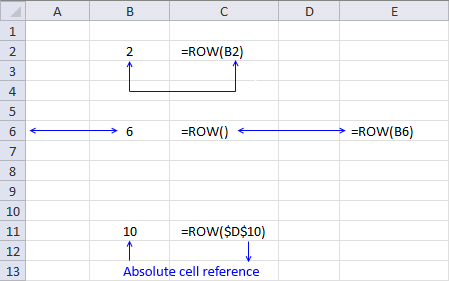You can enter a reference to a single cell or a cell range in the ROW function. Note that it will return an array of numbers if you enter a reference to a cell range, as long as you enter it as an array formula.

Formula in cell B2:

=ROW(B2)

B2 is a relative cell reference meaning it changes when you copy the cell and pastes it to another cell.

ROW(B2)

returns 2.

Formula in cell B6:

=ROW()

This example shows that you can omit the argument, the formula will default to the cell where the formula is entered.

The formula is entered in cell B6 in the example above, so it returns 6.

Formula in cell B11:

=ROW(\$D\$10)

\$D\$10 is an absolute cell reference meaning it won't change if you copy the cell and paste to other cells. Absolute and relative references in Excel

Note that an absolute cell reference will change if you add or delete rows above the cell.

returns 10.

The following example is not demonstrated in the image above. Type this formula in any cell:

=ROW(A2:A5)

To enter the formula as an array formula, follow these steps: Press and hold CTRL + SHIFT simultaneously, then press Enter. Release all keys.

The formula is now surrounded by curly brackets, like this: {=ROW(A2:A5)}. Don't enter these characters yourself, they appear automatically.

The cell will now only show the first value in the array which is 2, you must enter the array formula in a cell range equal of size as there are values in the array to be able to see them all.

Update - Dynamic arrays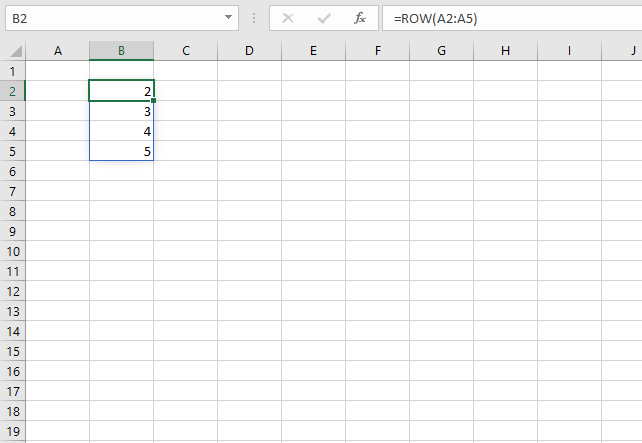Office 365 subscribers can now take advantage of dynamic arrays. They behave differently than regular array formulas, simply enter them as a regular formula and Excel will automatically extend the formula to cells below so you can see all values.

This behavior is called spilling, see image above. The cell range containing spilled values have a blue border, it will disappear as soon as you press with left mouse button on outside the cell range.

Microsoft recommends that you use dynamic arrays instead of regular array formulas, however, they are still working and compatible with older Excel versions.

## 4. Create an array of sequential numbers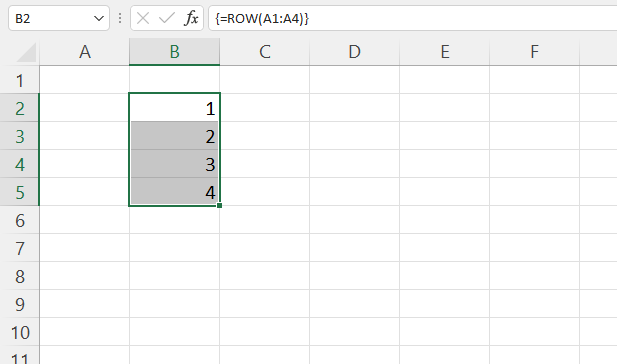The image above demonstrates a formula that creates an array of sequential numbers from 1 to 4. This can be used to number any cell range in order to get the appropriate values/rows.

Excel 365 subscribers can use the SEQUENCE function which offers more granular control. Most Excel 365 functions don't need the SEQUENCE function to number values/rows. For example, the FILTER function keeps track of values by itself.

Array formula in cell B2:

=ROW(A1:A4)

### 4.1 How to enter an array formula

1. Select cell range B2:B5 with your mouse.
2. Type: =ROW(A1:A4)
3. Press and hold CTRL + SHIFT keys simultaneously.
4. Press Enter once.
5. Release all keys.

The formula in the formula bar has a leading and ending curly bracket, they appear automatically when you follow all 5 steps above.

Don't enter these characters yourself.

## 5. Example 2 - Duplicate columns in an array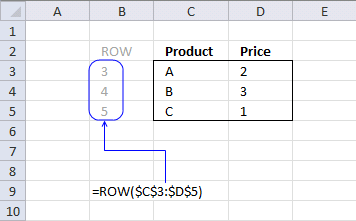This example demonstrates that if you provide a cell reference pointing to a cell range containing multiple columns the formula will still only return a single column of row numbers.

=ROW(\$C\$3:\$D\$5)

returns this array {3; 4; 5}.

The semicolon is a separating character telling you that the values are on a row each. A comma is used to separate values distributed across columns.

Now you might wonder why the array formula doesn´t return {3, 3; 4, 4; 5, 5}. There are six cells in the cell range, how come only three values are returned?

The answer is that there is no need for multiple duplicate columns in the array. Excel simplifies the array down to a single column.

But when used with multiple cell ranges in more complicated array formulas, make sure the number of rows matches.
See this example: Unique distinct values from a cell range

## 6. Example 3 - Number rows in any cell range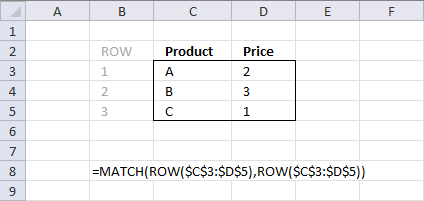The INDEX function returns a value of the cell at the intersection of a particular row and column, in a given range. To be able to work with multiple values from an arbitrary cell range using the INDEX function we must number each row.

That is exactly what we did in the previous example but the first row in a given range has to be 1 and the second 2 and so on. Why? The INDEX function needs properly numbered cell ranges. This is where the ROW and MATCH function comes in.

=MATCH(ROW(cell_range), ROW(cell_range))

The beauty with this formula is that it returns row numbers from any cell range no matter size or location.

Array formula in cell range B3:B5:

=MATCH(ROW(C3:D5), ROW(C3:D5))

becomes =MATCH({3; 4; 5}, {3; 4; 5}) and returns {1; 2; 3}. This array has the same number of values as there are rows in cell range C3:D5.

Remember, enter the formula as an array formula.

So what can you do with row numbered cell ranges?

The MATCH function can also be used to find the relative position of a value in a cell range. See these posts:

## 7. How to number rows?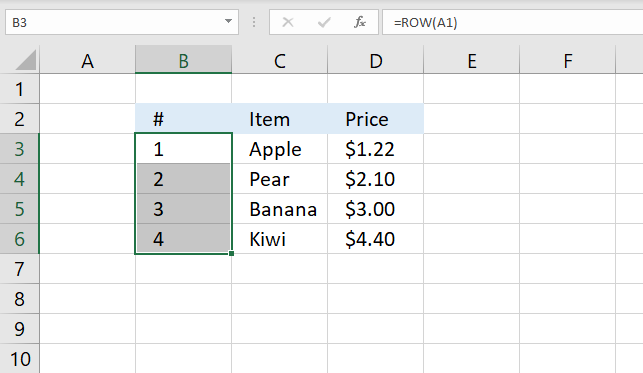The image above demonstrates how to number records in a data set, however, this method is not great and I will show you why.

Here are the steps:

1. Select cell B3.
2. Type: =ROW(A1)
3. Press Enter.
4. Copy cell B3.
5. Paste to cells below as far as needed.

Why not use this formula? The formula will break if you insert more rows above, the cell reference is relative meaning it will change. The same thing will happen with an absolute formula.

I recommend you use the ROWS function with an expanding cell reference.

Formula in cell B3:

=ROWS(\$A\$1:A1)

Copy cell B3 and paste to cells below, this will adjust the expanding cell reference accordingly. This will not break if you insert more rows.